# Texas Go Math Grade 4 Lesson 17.5 Answer Key Stem-and-Leaf Plots

Refer to our Texas Go Math Grade 4 Answer Key Pdf to score good marks in the exams. Test yourself by practicing the problems from Texas Go Math Grade 4 Lesson 17.5 Answer Key Stem-and-Leaf Plots.

## Texas Go Math Grade 4 Lesson 17.5 Answer Key Stem-and-Leaf Plots

Essential Question

How do you make stem-and-leaf plots with whole numbers?
stem-and-leaf plot is a way of organizing data into a form to easily look at the frequency of different types of values.
Constructing a Stemplot: In a stemplot, each score is broken into two pieces: the stem and leaf. In this example, the tens digits are stems, and the one digits form the leaves. The resulting stemplot produces a distribution of the data similar to a histogram, but all of the data values are retained in a compact form. You can easily see features of the student’s performance from the shape of the stem-and-leaf plot.
Stem and Leaf Plot Example: Suppose that your class had the following test scores: 84, 65, 78, 75, 89, 90, 88, 83, 72, 91, and 90 and you wanted to see at a glance what features were present in the data. You would rewrite the list of scores in order and then use a stem-and-leaf plot. The stems are 6, 7, 8, and 9, corresponding to the tens place of the data. This is listed in a vertical column. The one’s digit of each score is written in a horizontal row to the right of each stem, as follows:
9| 0 0 1
8| 3 4 8 9
7| 2 5 8
6| 2
You can easily read the data from this stemplot. For example, the top row contains the values of 90, 90, and 91. It shows that only three students earned a score in the 90th percentile with scores of 90, 90, and 91. By contrast, four students earned scores in the 80th percentile, with marks of 83, 84, 88, and 89.

Unlock the Problem

Henry kept track of the points of each of his words when he played a word game with his friend.Then he used a stem-and-leaf plot to show the data.

A stem-and-leaf plot shows groups of data arranged by place value.

Make a stem and leaf plot.

Step 1: Group the data by the tens of digits.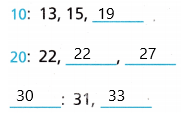STEP 2
Order the tens digits from least to greatest. Draw a line.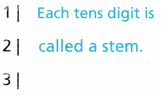1|
2|
3|
STEP 3STEP 4
Include a title, lables, and a key.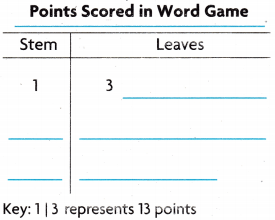1| 5 represents 15 points
1| 9 represents 19 points
2| 2 represents 22 points
2| 2 represents 22 points
2| 7 represents 27 points
3| 1 represents 31 points
3| 3 represents 33 points

Math Talk

Mathematical Processes
Explain how a stem-and-leaf, plot uses place value.
A Stem and Leaf plot shows a large amount of data in a clear way by listing it in order of place value. It is generally used when data has multi-digit numbers.

Share and Show

Question 1.
Use the data in the table to make a stem-and-leaf plot.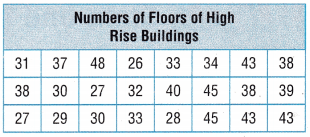Order the data in the table from ____________ to ____________.
The numbers ____________, ____________, ____________ are stems.
The leaves for stem 2 are ____________, ____________, ____________, ____________, ____________.
Complete the stem -and – leaf plot.
1. Determine the largest and smallest number:
Order the data in the table from lowest to greatest.
The numbers 2, 3, 4 are the stems.
The leaves for stem 2 are 6, 7, 7, 8, 9.
The leaves for stem 3 are 0, 0, 1, 2, 3, 3, 4, 7, 8, 8, 8, 9.
The leaves for stem 4 are 0, 3, 3, 3, 5, 5, 8
2. Draw the vertical line:
2|
3|
4|
3. Fill in the leaves: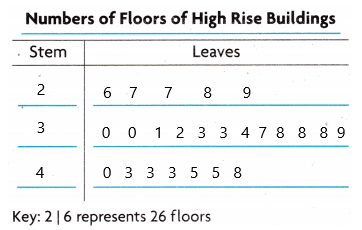2| 7 represents 27 floors
2| 7 represents 27 floors
2| 8 represents 28 floors
2| 9 represents 29 floors
3| 0 represents 30 floors and so on… Likewise need to write all the keys.

Use the data in the Number of Jumps table to make a stern-and-leaf plot.1. Determine the largest and smallest number:
Order the data in the table from lowest to greatest.
The numbers 1, 2, 3 are the stems.
The leaves for stem 1 are 0, 1, 2.
The leaves for stem 2 are 0, 2, 5, 6.
The leaves for stem 3 are 1.
2. Draw the vertical line:
1|
2|
3|
3. Fill in the leaves: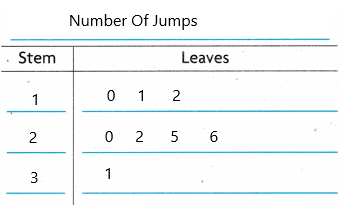1| 0 represents 10 jumps
1| 1 represents 11 jumps
1| 2 represents 12 jumps
2| 0 represents 20 jumps
2|2 represents 22 jumps
2| 5 represents 25 jumps
2| 6 represents 26 jumps
3| 1 represents 31 jumps.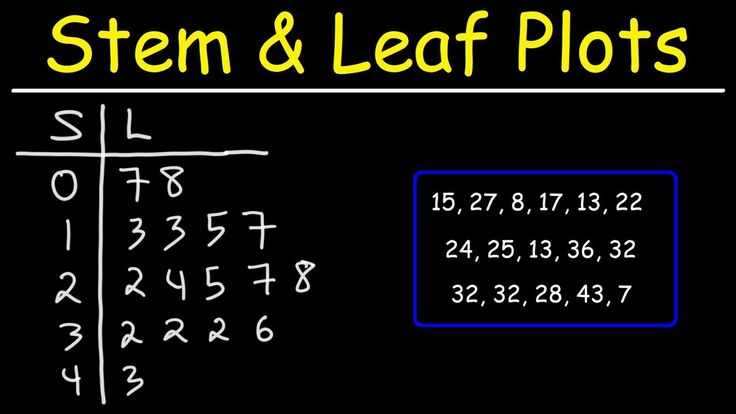Question 3.
Use the data in the Number of Different Beads table to make a stem-and-leaf plot.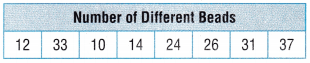1. Determine the largest and smallest number:
Order the data in the table from lowest to greatest.
The numbers 1, 2, 3 are the stems.
The leaves for stem 1 are 0, 2, 4.
The leaves for stem 2 are 4, 6.
The leaves for stem 3 are 1, 3, 7.
2. Draw the vertical line:
1|
2|
3|
3. Fill in the leaves:Problem Solving

Question 4.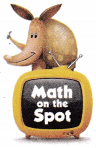H.O.T. Mike records his bowling scores and puts them in a table. He wants to easily see how many times he bowled in the 90s. Make a stem-and-leaf plot from the data in the table. Explain how Mike used the stein-and-leaf plot to determine how many times he bowled in the 90s.1. Determine the largest and smallest number:
Order the data in the table from lowest to greatest.
The numbers 6, 7, 8, 9 are the stems. But in the question only asked about the ’90s.
The leaves for stem 6 are 1, 7, 9.
The leaves for stem 7 are 3, 3, 4, 5, 6, 6, 9.
The leaves for stem 8 are 0, 1, 2, 5, 5, 6, 6, 6, 9.
The leaves for stem 9 are 2, 2, 3, 4, 8
2. Draw the vertical line:
6|
7|
8|
9|
3. Fill in the leaves:Now I am writing only 90’s:
9| 2 represents 92 bowling.
9| 2 represents 92 bowling.
9| 3 represents 93 bowling.
9| 4 represents 94 bowling.
9| 8 represents 98 bowling.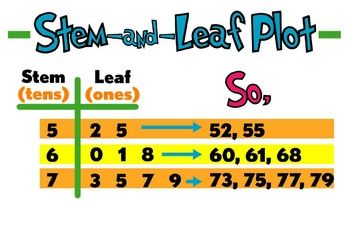Texas Go Math Grade 4 Stem and Leaf Plot Answer Key Question 5.
Use Graphs Multi-Step Naomi conducted a science experiment where she recorded the high temperature each day for 24 days. She chose a stem-and-leaf plot to display her data. Make Naomi’s stem-and-leaf plot.1. Determine the largest and smallest number:
Order the data in the table from lowest to greatest.
The numbers 6, 7, 8 are the stems.
The leaves for stem 6 are 2, 5, 7, 7, 8.
The leaves for stem 7 are 2, 2, 2, 3, 5, 5, 6, 9, 9.
The leaves for stem 8 are 3, 4, 5, 6, 6, 6, 7, 7, 8, 9.
2. Draw the vertical line:
6|
7|
8|
3. Fill in the leaves: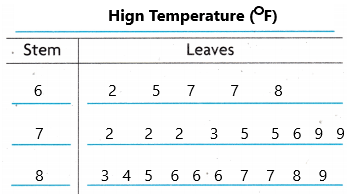6| 2 represents 62 temperature.
6| 5 represents 65 temperature.
6| 7 represents 67 temperature.
6| 7 represents 67 temperature.
6| 8 represents 68 temperature.
7| 2 represents 72 temperature.
7| 2 represents 72 temperature.
7| 2 represents 72 temperature.
7| 3 represents 73 temperatures.
7| 5 represents 75 temperature.
7| 5 represents 75 temperature.  And so on… continue up to 8| 9.

Question 6.
H.O.T. Use Graphs Multi-Step Jenny was asked to make teams based on the height of the students in her class. She recorded the student’s height, in inches, in a table. To more clearly see the different heights, she made a stem-and-leaf plot. Make Jenny’s stein-and-leaf plot.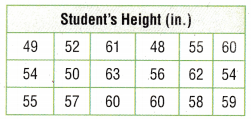1. Determine the largest and smallest number:
Order the data in the table from lowest to greatest.
The numbers 4, 5, 6 are the stems.
The leaves for stem 4 are 8, 9.
The leaves for stem 5 are 0, 2, 4, 4, 5, 5, 6, 7, 8, 9.
The leaves for stem 6 are 0, 0, 0, 1, 2, 3.
2. Draw the vertical line:
4|
5|
6|
3. Fill in the leaves: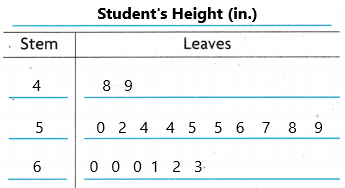4| 8 represents 48 height.
4| 9 represents 49 height.
5| 0 represents 50 height.
5| 2 represents 52 height.
5| 4 represents 54 height and so on… write all the keys up to 6| 3.

Stem and Leaf Plot 4th Grade Go Math Answer Key Question 7.
H.O.T. Use Math Language Explain the steps you would use to make the stem-and-leaf plot from the data in the table. Then make the stem-and-leaf plot.1. Determine the largest and smallest number:
Order the data in the table from lowest to greatest.
The numbers 2, 3, 4, 5 are the stems.
The leaves for stem 2 are 2, 4, 4, 6, 9, 9.
The leaves for stem 3 are  0, 0, 2, 5, 6, 7.
The leaves for stem 4 are 1, 1, 4, 7.
The leaves for stem 5 are 5, 5.
2. Draw the vertical line:
2|
3|
4|
5|
3. Fill in the leaves:2| 2 represents 22 minutes.
2| 4 represents 24 minutes.
2| 4 represents 24 minutes.
2| 6 represents 26 minutes.
2| 9 represents 29 minutes.
2| 9 represents 29 minutes and so on… write the keys up to 5| 5.

Use the table for 8-11.

The data shows the number of jumping jacks completed by 15 students in one minute. Robert is making a stem-and-leaf plot to display the information.Question 8.
What are the stems for the stem-and-leaf plot?
(A) 3, 5, 6
(B) 0, 3, 4, 5, 9
(C) 3, 4, 5
(D) 0, 1, 2, 3, 5, 8, 9Explanation:
1. Determine the largest and smallest number:
Order the data in the table from lowest to greatest.
The numbers 3, 4, 5 are the stems.
2. Draw the vertical line:
3|
4|
5|
Question 9.
How many leaves are in the stem-and-leaf plot?
(A) 12
(B) 10
(C) 15
(D) 3Explanation:
Count all the leaves. There are 15 leaves.
Question 10.
Which could be a key for the stem-and-leaf plot?
(A) 4 | 8 represents 84 jumping jacks.
(B) 3 | 8 represents 38 jumping jacks.
(C) 5 | 0 represents 5 jumping jacks.
(D) 4 | 4 represents 4 jumping jacks.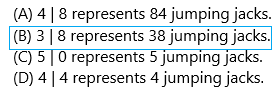Explanation:
Option B represents the correct.

TEX Test Prep

Texas Go Math Grade 4 Answer Key Stem and Leaf Plot Question 11.
In the stem-and-leaf plot that Robert is making, which stem would have the most leaves?
(A) 3
(B) 4
(C) 5
(D) 0Explanation: count all the 50’s: 50, 50, 51, 51, 52, 53, 55, 55.
The numbers 3, 4, 5 are the stems.
3|
4|
5|
For stem 3 the leaves are 8, 9.
For stem 4 the leaves are 2, 4, 4, 5, 8
For stem 5 the leaves are:0, 0, 1, 1, 2, 3, 5, 5.

### Texas Go Math Grade 4 Lesson 17.5 Homework and Practice Answer Key

Question 1.
Use the data in the Daily Temperatures table to make a stein-and-leaf plot.1. Determine the largest and smallest number:
Order the data in the table from lowest to greatest.
The numbers 7, 8, 9 are the stems.
The leaves for stem 7 are 9.
The leaves for stem 8 are 4, 8.
The leaves for stem 9 are 1, 2, 5, 5, 6.
2. Draw the vertical line:
7|
8|
9|
3. Fill in the leaves: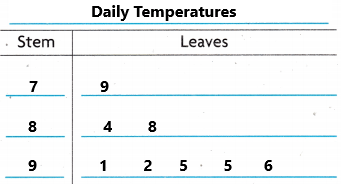7| 9 represents 79 temperature.
8| 4 represents 84 temperature.
8| 8 represents 88 temperature.
9| 1 represents 91 temperatures.
9| 2 represents 92 temperatures.
9| 5 represents 95 temperatures.
9| 5 represents 95 temperatures.
9| 6 represents 96 temperatures.

Question 2.
Use the data in the Minutes Spent Doing Homework table to make a stem-and-leaf plot.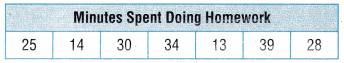1. Determine the largest and smallest number:
Order the data in the table from lowest to greatest.
The numbers 1, 2, 3 are the stems.
The leaves for stem 1 are 3, 4.
The leaves for stem 2 are 5, 8.
The leaves for stem 3 are 0, 4, 9.
2. Draw the vertical line:
1|
2|
3|
3. Fill in the leaves:1| 3 represents 13 minutes.
1| 4 represents 14 minutes.
2| 5 represents 25 minutes.
2| 8 represents 28 minutes.
3| 0 represents 30 minutes.
3| 4 represents 34 minutes.
3| 9 represents 39 minutes.

Problem Solving

Question 3.
Cade recorded the number of points his team scored in ten basketball games and put them in a table. Make a stem-and-leaf plot from the data.1. Determine the largest and smallest number:
Order the data in the table from lowest to greatest.
The numbers 2, 3 are the stems.
The leaves for stem 2 are 4, 5, 5, 6, 8, 8.
The leaves for stem 3 are 0, 2, 2, 4.
2. Draw the vertical line:
2|
3|
3. Fill in the leaves:2| 4 represents 24 points.
2| 5 represents 25 points.
2| 5 represents 25 points.
2| 6 represents 26 points.
2| 8 represents 28 points.
2| 8 represents 28 points.
3| 0 represents 30 points.
3| 2 represents 32 points.
3| 2 represents 32 points.
3| 4 represents 34 points.

Stem and Leaf Plot Worksheet with Answers Pdf Question 4.
The school librarian recorded the total number of books checked out from the library each day for two weeks. She put the data in a table. Make a stern-and-leaf plot from the data.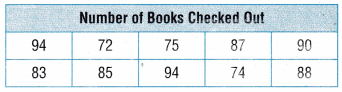1. Determine the largest and smallest number:
Order the data in the table from lowest to greatest.
The numbers 7, 8, 9 are the stems.
The leaves for stem 7 are 2, 4, 5.
The leaves for stem 8 are 3, 5, 7, 8.
The leaves for stem 9 are 0, 4, 4.
2. Draw the vertical line:
7|
8|
9|
3. Fill in the leaves:7| 2 represents 72 books.
7| 4 represents 74 books.
7| 5 represents 75 books.
8| 3 represents 83 books.
8| 5 represents 85 books.
8| 7 represents 87 books.
8| 8 represents 88 books.
9| 0 represents 90 books.
9| 4 represents 94 books.
9| 4 represents 94 books.

Lesson Check

Use the table at right for 5-7.

The data show the number of hours 16 students exercise in one month. Jen is making a stem-and-leaf plot to display the information.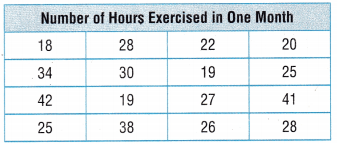Question 5.
What are the stems for the stem-and-leaf plot?
(A) 0, 1, 2, 4, 5, 6, 7, 8, 9,
(B) 0, 1, 2, 3, 4
(C) 1, 2, 3, 4
(D) 2, 3, 4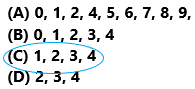Explanation:
1. Determine the largest and smallest number:
Order the data in the table from lowest to greatest.
The numbers 1, 2, 3, 4 are the stems.
Question 6.
Which stem has the most leaves?
(A) 1
(B) 2
(C) 3
(D) 4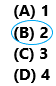Explanation:
1. Determine the largest and smallest number:
Order the data in the table from lowest to greatest.
The numbers 1, 2, 3, 4 are the stems.
The leaves for stem 1 are 8, 9, 9.
The leaves for stem 2 are 0, 2, 5, 5, 6, 7, 8, 8.
The leaves for stem 3 are 0, 4, 8.
The leaves for stem 4 are 1, 2.

Go Math Answer Key 4th Grade Stem and Leaf Plot Practice Question 7.
Multi-Step How many more leaves are there for 2 than there are for 3 and 4 combined?
(A) 5
(B) 2
(C) 3
(D) 1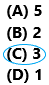Explanation:
1. Determine the largest and smallest number:
Order the data in the table from lowest to greatest.
The numbers 1, 2, 3, 4 are the stems.
The leaves for stem 1 are 8, 9, 9.
The leaves for stem 2 are 0, 2, 5, 5, 6, 7, 8, 8.
The leaves for stem 3 are 0, 4, 8.
The leaves for stem 4 are 1, 2.
The number of more leaves are there for 2 than there are for 3 and 4 combined: 8-5=3.

Use the table at right for 8-10

The data show the height of plants grown for Javier’s science experiment. Javier is making a stem-and-leaf plot to display the information.Question 8.
How many leaves are in the stem-and-leaf plot?
(A) 2
(B) 12
(C) 9
(D) 10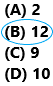Explanation:
count all the leaves present in the table.

Question 9.
Which could be a key for the stem-and-leaf plot?
(A) 2|2 represents 22 inches.
(B) 3|2 represents 23 inches.
(C) 2|1 represents 12 inches.
(D) 2|0 represents 2 inches.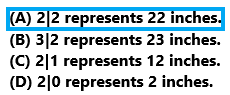Question 10.
Multi-Step How many leaves are in the plot for 12 and 14?
(A) 3
(B) 1
(C) 2
(D) 5